semPaths {semPlot} R Documentation

Plot path diagram for SEM models.

Description

This function creates a path diagram of a SEM model (or general linear model), which is then plotted using `qgraph`. Currently many different SEM programs and packages are supported. Please see my website (www.sachaepskamp.com) for more details on which packages are supported and what is supported for each package.

Usage

```semPaths(object, what = "paths", whatLabels, style, layout = "tree",
intercepts = TRUE, residuals = TRUE, thresholds = TRUE,
intStyle = "multi", rotation = 1, curve, nCharNodes = 3,
nCharEdges = 3, sizeMan = 5, sizeLat = 8, sizeInt = 2, ask,
mar, title, title.color = "black", include,
combineGroups = FALSE, manifests, latents, groups, color,
residScale, gui = FALSE, allVars = FALSE, edge.color,
reorder = TRUE, structural = FALSE, ThreshAtSide = FALSE,
threshold.color, fixedStyle = 2, freeStyle = 1,
as.expression, optimizeLatRes = FALSE, mixCols = TRUE,
curvePivot, ...)

```

Arguments

 `object` A `"semPlotModel"` object or any of the input types that can be used in `semPlotModel` directly. `what` What should the edges indicate in the path diagram? This function uses `grepl` to allow fuzzy matching and is not case sensitive. E.g., `par` will also match `Parameters`. `path`, `diagram` or `mod`This will display the model as an unweighted network (gray edges by default). `est` or `par`This will display the parameter estimates as weighted edges. `stand` or `std`This will display the standardized parameter estimates, if available, as weighted edges. `eq` or `cons`This is the same graph as `path`. except that parameters with equality constraints are now colored. Parameters with the same color are constrained to be equal. `col`This will create an unweighted graph of the path diagram, where edges are colored with a mix of the colors of connected nodes. `whatLabels` What should the edge labels indicate in the path diagram? This function uses `grepl` to allow fuzzy matching and is not case sensitive. E.g., `par` will also match `Parameters`. Default depends on the `what` argument, defaulting to the respective elements in the list below for values of `what` in the list above. `path`, `diagram`, `model`, `name` or `label`This will display the edge names as labels. `est` or `par`This will display the parameter estimate in edge labels. `stand` or `std`This will display the standardized parameter estimate in edge labels. `eq` or `cons`This will display the parameter number in edge labels. 0 indicates the parameter is fixed, parameters with the same parameter number are constrained to be equal. `no`, `omit`, `hide` or `invisible`Hides edge labels. `style` The style to use. Currently only indicates what the (residual) variances look like. Use `"mx"` or `"OpenMx"` for double headed selfloops and `"lisrel"` for single headed edges with no node as origin. `layout` A string indicating how the nodes should be placed. Similar to the 'layout' argument in `qgraph`. Can be one of the following strings. treeThe integrated tree-like layout. Places exogenous variables at the top and endogenous variables at the bottom. See 'details' for more details. circleThe same layout as "tree", except that afterwards the horizontal levels of the layout are placed in circles. Especially useful for models with a large number of manifest variables and a relatively small number of latent variables. springCalls the "spring" layout in `qgraph`, which uses the Fruchterman-reingold algorithm (Fruchterman & Reingold, 1991). tree2Calls the `layout.reingold.tilford` function from the igraph package (Csardi & Nepusz, 2006), which uses the Reingold-Tilford algorithm (Reingold & Tilford, 1981). Before calling the algorithm roots are chosen and a slightly modified version of the graph is used to produce consistent results. See 'details'. circle2The same layout as "tree2", except that afterwards the horizontal levels of the layout are placed in circles. Other optionsIf the assigned value is not in this list it is sent to `qgraph`. This allows for manual specification of the layout as well as using functions found in the 'igraph; library. `intercepts` Logical, should intercepts be included in the path diagram? `residuals` Logical, should residuals (and variances) be included in the path diagram? `thresholds` Logical, should thresholds be included in the path diagram? `intStyle` Style of the intercepts. `"multi"` plots a separate unit vector node for each intercept and `"single"` plots a single unit vector node. Currently, `"single"` is not well supported and might lead to unexpected results. `rotation` An integer indicating the rotation of the layout when "tree" or "tree2" layout is used. 1, 2, 3 and 4 indicate that exogenous variables are placed at the top, left side, bottom and right side respectively. `curve` The curvature of the edges. In tree layouts this argument only curves the edges that are between nodes on the same level. e.g., correlations between exogenous manifest variables. `nCharNodes` Number of characters to abbreviate node labels to (using `abbreviate`). Set to 0 to omit abbreviation. `nCharEdges` Number of characters to abbreviate edge labels to (using `abbreviate`). Set to 0 to omit abbreviation. `sizeMan` Size of the manifest nodes, sent to the 'vsize' argument in `qgraph`. `sizeLat` Size of the latent nodes, sent to the 'vsize' argument in `qgraph`. `sizeInt` Size of the unit vector nodes, sent to the 'vsize' argument in `qgraph`. `ask` Specifies the 'ask' parameter in `par`. Defaults to TRUE if multiple groups are in the model. `mar` Same as the 'mar' argument in `qgraph`. By default this argument is based on the values of 'rotation', 'style' and 'title'. `title` Logical, should titles be plotted of the group names above each plot? `title.color` Color of the titles. `include` Integer vector indicating which groups should be included in the output. e.g., to only plot a diagram for the first group use `include = 1`. `combineGroups` Logical. If TRUE all groups are combined in the same path diagram. `manifests` A character vector in which every element is the name of a manifest variable in the model. This argument can be used to overwrite the order in which nodes are plotted in the graph if `reorder = FALSE` `latents` A character vector in which every element is the name of a latent variable in the model. This argument can be used to overwrite the order in which nodes are plotted in the graph if `reorder = FALSE` `groups` Groups nodes that should be colored the same, similar to the 'groups' argument in `qgraph` with a few exceptions. Should be a list containing in each element the names (instead of numbers as in qgraph) of nodes that belong together. Nodes that are indicated to belong to a group will be assigned the same color, as given by the 'color' argument. Nodes not belonging to a group will be assigned the color "", which indicates that they will inherit a mix of the colors of connected nodes (or white, if no connected nodes are colored.) In addition, this argument can be assigned a single character: "manifests", "latents" or "both" to make a single group for each manifest, latent or both manifest and latent variables. e.g., `groups = "latents"` will color each latent variable uniquely, and color all manifest variables a mixture of the colors of latents they load on. `color` Controls the color of nodes. Similar to 'color' in `qgraph`. A color vector indicating the color for each group, a single color character indicating the color for all nodes or a color vector indicating the color for each node separately. Can also be a list contaning one or more of the following elements (using fuzzy matching): manThe colors for manifest nodes latThe colors for latent nodes intThe color for intercepts `residScale` The size of residual edges if `style = "lisrel"`. Defaults to two times the value of 'sizeMan'. `gui` Not yet implemented. `allVars` Logical. If TRUE all variables are plotted in the path diagrams for each group. If FALSE only variables are plotted that are used in the group. `edge.color` A value indicating the color of all edges or a vector indicating the color of each edge. Useful for manually overwriting edge colors. `reorder` Logical. Should manifest variables be reordered to be near latent factors they are most connected to in the "tree" layout? If FALSE manifest variables are placed in the order they appear in the RAM. `structural` Logical. Set this to FALSE to only show the structural model (omit all manifest variables.) `ThreshAtSide` Logical. If TRUE, thresholds are plotted as small lines at the side of manifest nodes, otherwise they are plotted as lines inside the nodes. `threshold.color` Color of the threshold lines. Defaults to "black" `fixedStyle` A vector of length one or two specifying the color and line type (same as 'lty' in `par`) of fixed parameters. Can be both character and numeric. If one of the elements encodes a color it is used to overwrite the color of fixed edges, and if an element can be coerced to a numeric it is used to encode the line type. For example, ` fixedStyle = c("red",3)` specifies that all fixed parameters should be visualized with a red edge with `lty=3` `freeStyle` Same as 'fixedStyle' but for free parameters instead. `as.expression` A character vector indicating which labels should be treated as an `expression`, so that mathematical notation and Greek letters can be used in the path diagram. If this vector contains `"nodes"` all node labels are converted to expressions, and if this vector contains `"edges"` all node labels are converted to expressions. `optimizeLatRes` Logical. If this is TRUE, the angle of the incoming residuals on latent variables is attempted to be optimally chosen so its position conflicts with the least amount of connected edges. `mixCols` Logical, should uncolored nodes obtain a mix of connected colored nodes? Defaults to `TRUE`. `curvePivot` Same as the `curvePivot` argument in `qgraph`. Defaults to `TRUE` in tree layouts and FALSE otherwise. `...` Arguments sent to the `qgraph` function.

Details

The default `"tree"` layout under default rotation places the nodes in one of four horizontal levels. At the top the exogenous manifest variables, under that the exogenous latent variables, under that the endogenous latent variables and at the bottom the endogenous manifest variables. If one of these kinds of variables does not exist its level is omitted. Afterwards, the `rotation` argument will rotate the graph and the `"circle"` layout will make the layout circular using these levels as nested circles.

If not manually set (see `semPlotModel-edit`), `semPath` will automatically try to set the endogenous and exogenous variables, such that the resulting layout looks good. A latent variable is identified as exogenous if it is not on the right hand side of a directed edge (`->` or `~>`) with another latent variable as node of origin. A manifest variable is set as exogenous if it is only connected, in any way, to exogenous latent variables and if it is not the right hand side (dependent variable) of a regression edge (`~>`). If all variables are set to exogenous this way, they are all set to endogenous for consistency in the layouts. Afterwards, manifest variables only used in formative measurement models (only outgoing directed edges to latents) are set to exogenous again so that MIMIC models are displayed properly.

Intercepts are placed on the same level as the variable, either on the left or right side of the node (pointing outward from the center). Residuals for manifest variables are placed at the top or bottom (for exogenous and endogenous manifests respectively). Residuals of latents are placed at the bottom or top respectively for exogenous and endogenous variables, but is switched if the latent is not connected to a manifest. Residuals for the leftmost and rightmost latent are placed at the left and right side respectively, or diagonal if the latent is connected to an intercept.

The `"tree2"` and `"circle2"` layouts call the `layout.reingold.tilford` function from the `igraph` package. As roots are used the first available variables of the following list:

• Intercepts of exogenous manifests

• Exogenous manifest

• Intercepts of exogenous latents

• Exogenous latents

• Interceots of endogenous latents

• Endogenous latents

• Intercepts of endogenous manifests

• The endogenous manifest with the most outgoing edges (this should not be possible by default, but can be manually set)

• The most connected endogenous manigest.

To compute an optimal layout `layout.reingold.tilford` is run on a slightly altered version of the path diagram. In this version, the direction of edges from all intercepts that are not roots is reversed, the direction of all edges leading to exogenous manifests is reversed and all bidirectional edges are removed.

Value

A `"qgraph"` object as returned by `qgraph`. This object can be used to alter the graph (such as manually redefining the layout) and to plot the graph again with different arguments.

If there are multiple groups a list is returned with a "qgraph" object for each path diagram that has been produced.

Author(s)

Sacha Epskamp <mail@sachaepskamp.com>

References

Fruchterman, T. & Reingold, E. (1991). Graph drawing by force-directed placement. Software - Pract. Exp. 21, 1129-1164.

Reingold, E and Tilford, J (1981). Tidier drawing of trees. IEEE Trans. on Softw. Eng., SE-7(2):223-228.

Csardi G, Nepusz T (2006). The igraph software package for complex network research, InterJournal, Complex Systems 1695. http://igraph.sf.net

`qgraph` `semPlotModel` `semPlotModel-class` `semCors` `lisrelModel` `semSyntax`

Examples

`````` # Regression analysis with interaction effects ----------------------------

# A silly dataset:
X <- rnorm(100)
Y <- rnorm(100)
Z <- rnorm(1)*X + rnorm(1)*Y + rnorm(1)*X*Y
DF <- data.frame(X,Y,Z)

# Regression including interaction:
res <- lm(Z ~ X*Y, data = DF)

# Path diagram:
semPaths(res)
``````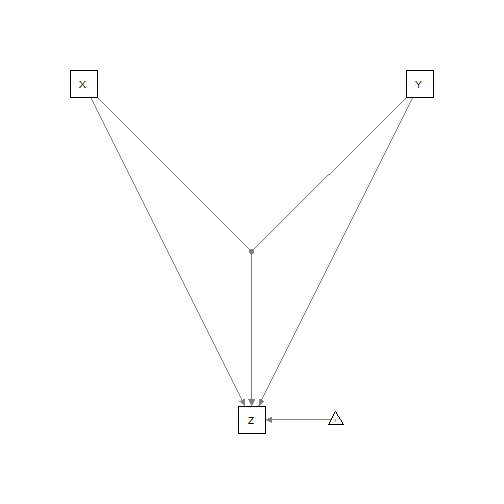``````
# Standardized estimates:
semPaths(res,"std","hide")
``````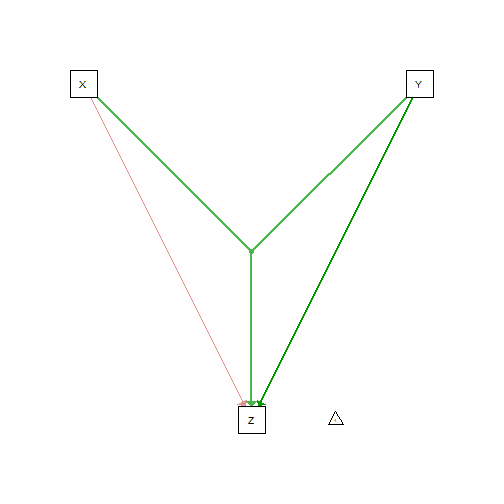``````

# MIMIC model ----------------------------------------------------
## Lavaan

library("lavaan")

# Example 5.8 from mplus user guide:
names(Data) <- c(paste("y", 1:6, sep=""),
paste("x", 1:3, sep=""))

# Model:
model.Lavaan <- 'f1 =~ y1 + y2 + y3
f2 =~ y4 + y5 + y6
f1 + f2 ~ x1 + x2 + x3 '

# Run Lavaan:
library("lavaan")
fit <- lavaan:::cfa(model.Lavaan, data=Data, std.lv=TRUE)

# Plot path diagram:
semPaths(fit,title=FALSE, curvePivot = TRUE)
``````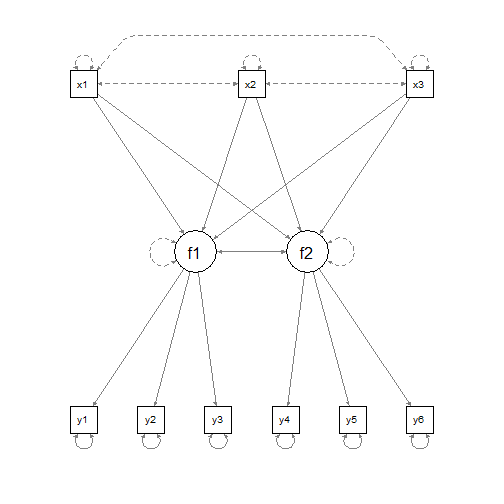``````
# Standardized parameters:
semPaths(fit,"std",edge.label.cex=0.5, curvePivot = TRUE)
``````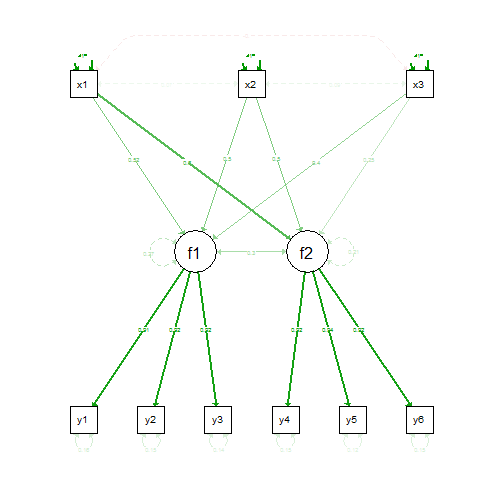``````

## Mplus

# Same model, now using mplus output:

# Plot model:
semPaths(outfile,intercepts=FALSE)
``````
``````## Reading model:  C:\Users\Sacha\AppData\Local\Temp\Rtmp862YCS\file6106f6e46ec.out
``````
``````## Loading required package: gsubfn
``````
``````## Loading required package: proto
``````
``````## Loading required package: plyr
``````
``````## Loading required package: coda
``````
``````## Loading required package: lattice
``````
``````## Attaching package: 'lattice'
``````
``````## The following object(s) are masked from 'package:boot':
##
## melanoma
``````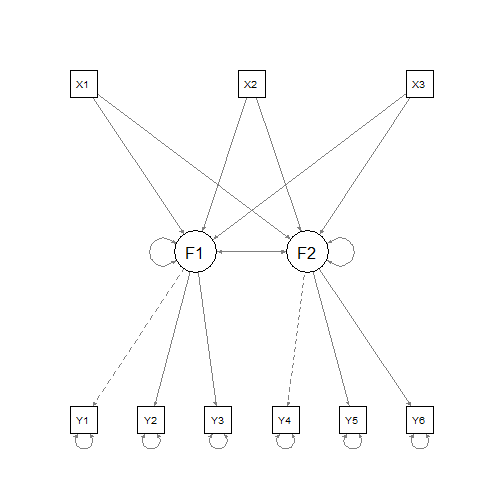``````# Note that mplus did not report the fixed variances of the exogenous variables.

# Thresholds  -----------------------------------------------------
## Lavaan

# Example 5.8 from mplus user guide:
names(Data) <- c("u1","u2","u3","u4","u5","u6")
Data <- as.data.frame(lapply(Data, ordered))

# Lavaan model:
model <- ' f1 =~ u1 + u2 + u3; f2 =~ u4 + u5 + u6 '

# Run Lavaan:
fit <- lavaan::cfa(model, data=Data)

# Plot path diagram:
semPaths(fit,intercepts=FALSE)
``````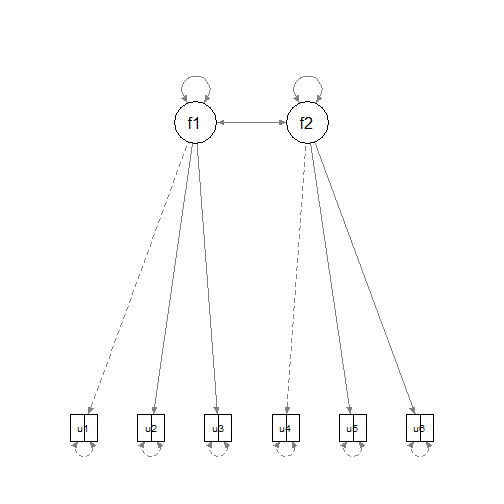``````

## Mplus

# Same model, now using mplus output:

# Plot model:
semPaths(outfile)
``````
``````## Reading model:  C:\Users\Sacha\AppData\Local\Temp\Rtmp862YCS\file6102e2914f5.out
``````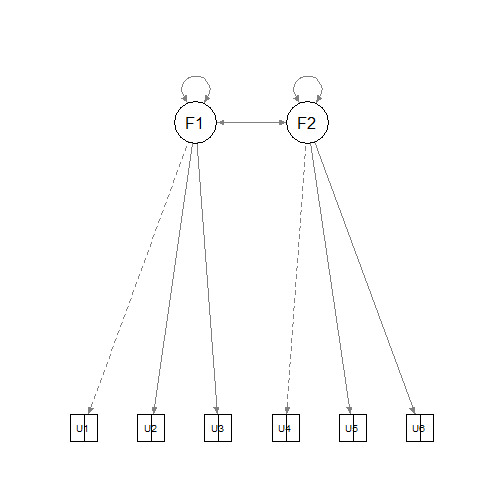``````

# OpenMx ----------------------------------------------------------
## Not run:
##D # To isntall OpenMx see:
##D # http://openmx.psyc.virginia.edu/
##D
##D library("OpenMx")
##D
##D # Example from mxRun help page:
##D # Create and run the 1-factor CFA on the openmx.psyc.virginia.edu front page
##D data(demoOneFactor)  # load the demoOneFactor dataframe
##D manifests <- names(demoOneFactor) # set the manifest to the 5 demo variables
##D latents   <- c("G")  # define 1 latent variable
##D model <- mxModel("One Factor", type="RAM",
##D   manifestVars = manifests,
##D     latentVars   = latents,
##D     mxPath(from=latents  , to=manifests),
##D     mxPath(from=manifests, arrows=2),
##D     mxPath(from=latents  , arrows=2, free=FALSE, values=1.0),
##D     mxData(cov(demoOneFactor), type="cov", numObs=500)
##D )
##D model <- mxRun(model) #run model, returning the result
##D
##D # Plot with colors from OpenMx front page:
##D semPaths(model, color = list(
##D               lat = rgb(245, 253, 118, maxColorValue = 255),
##D               man = rgb(155, 253, 175, maxColorValue = 255)),
##D           mar = c(10, 5, 10, 5))
##D
##D ## Factor Analysis:
##D source("http://openmx.psyc.virginia.edu/svn/trunk/demo/TwoFactorModel_PathCov.R")
##D semPaths(twoFactorFit, layout = "tree2")
##D
##D
##D
##D
##D
##D # Multi-group analysis -------------------------------------------
##D ## LISREL: